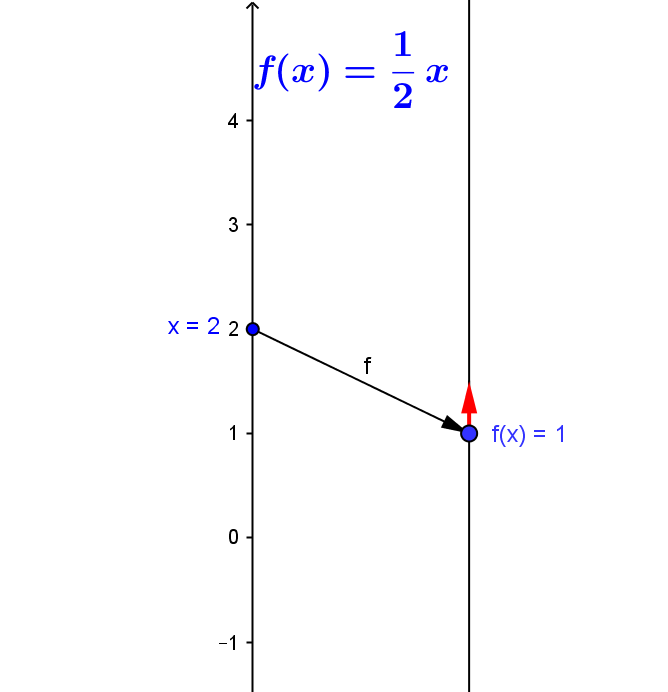Definition: We say that a  $f$  is (montonically)  increasing for a set  D, if whenever $a < b$ for $a$ and $b \in D$ , $f(a) < f(b)$.
We say that a  $f$  is (montonically)  decreasing if whenever $a < b$ for $a$ and $b \in D$, $f(a) > f(b)$.
Definition: We say that a  $f$  is monotonic for a set  D, if either $f$ is (monotically) increasing or $f$  is (monotonically)  decreasing for the set $D$.
 Graph Mapping Diagram Increasing: If $a < b$ then $f(a) < f(b)$.Decreasing: If $a < b$ then $f(a) > f(b)$.Definition: We say that a  $f$  is (monotonically) increasing or decreasing at a number or point $c$, if either $f$ is (monotonically) increasing or $f$  is (monotonically)  decreasing for an open interval $D = (a,b)$ with $c \in D$.

Mapping Diagram Visualization:
An arrow (pointing up or down) on the target axis at the point representing the value of the function at $c$, $f(c)$, can be used to indicate whether $f$ is increasing (pointing up) or decreasing (pointing down) at $c$.
When appropriate the magnitude of such a target axis arrow can indicate the rate of increase  or decrease of the function values.

Example:
Consider $y = f(x) = \frac 12 x$. Then $f$ is increasing for $(-\infty, \infty)$. So $f$ is increasing at any number $x$.
Using $\frac 12$ as the rate of increase of the function values, the following mapping diagram visualizes this information.When $x=2$, the arrow from $x=2$ on the domain axis to $f(x)=f(2)=\frac 12 (2) = 1$ on the target axis shows the function connection.
The arrow pointing up on the target axis at $f(x)=1$ with length $\frac 12$ indicates that when $x=2$ the function $f$ is increasing at a rate of $\frac 12$ units of $y$ per unit of $x$    .